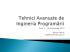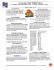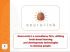# JCPS Grade 6- KCAS Curriculum Resource Map

## Transcription

JCPS Grade 6- KCAS Curriculum Resource Map
```JCPS Grade 6- KCAS Curriculum Resource Map
Weeks 6-17 Unit 2: Rational Number
48 instructional days + 12 additional days that could be used for assessment, intervention, and enrichment
Standards with red keys are priority standards.
Standard
Lessons
Teacher Notes
6.NS.4 Find the greatest common factor of two whole numbers less than or
CMP2: Prime Time
KCAS Notes: 6.NS.4 Be deliberate about
equal to 100 and the least common multiple of two whole numbers less
Investigation 2.3 & 3
representing sum of two whole numbers
than or equal to 12. Use the distributive property to express a sum of two
with a common factor using the distributive
whole numbers 1-100 with a common factor as a multiple of a sum of two
property. Also teachers should be
whole numbers with no common factor. For example, express 36 + 8 as
intentional about teaching GCF and LCM.
FORMATIVE
4(9+2).
1
ASSESSMENTS
Learning Targets:
 I can determine the Greatest Common Factor of two whole numbers less
than or equal to 100.
 I can determine the Least Common Multiple of two whole numbers less
than or equal to 12.
 I can apply the Distributive Property to rewrite addition problems by
factoring out the Greatest Common Factor of two whole numbers 1-100
and multiplying the Greatest Common Factor by the sum of the two
remaining factors.
6.EE.1 Write and evaluate numerical expressions involving whole-number
exponents.
Learning Targets:
 I can write numerical expressions involving whole number exponents
 I can evaluate numerical expressions involving whole number exponents.
6.NS.1 Interpret and compute quotients of fractions, and solve
word problems involving division of fractions by fractions, e.g., by
using visual fractions models and equations to represent the
problem. For example, create a story context for (2/3) ÷ (3/4) and use a
visual fraction model to show the quotient; use the relationship between
multiplication and division to explain that (2/3) ÷ (3/4) = 8/9 because 3/4 of
8/9 is 2/3. (In general, (a/b) ÷ (c/d) - ad/bc). How much chocolate will each
person get if 3 people share 1/2 lb of chocolate equally? How many 3/4-cup
servings are in 2/3 of a cup of yogurt? How wide is a rectangular strip of
Recommended Assessments
FA Opportunity
6.RP.3c
FA Opportunity
6.NS.1
FA Opportunity
6.NS.2
FA Opportunity
6.NS.5
FA Opportunity
6.NS.8
Weeks 6-17 Sample Unit
Resource Plan
2
Vocabulary: algebraic expressions,
absolute value, distributive property,
equivalent ratios, greatest common
factor, inequality, integer, least
common multiple, negative number,
opposite, percent, positive number,
rational number, solution
CMP2: Bits & Pieces II
Investigation 4
2
Analytical an Applied Sciences
KAG: MS Math 2012-13
*Standard Progression/CMP2 Alignment Strength
1
JCPS Grade 6- KCAS Curriculum Resource Map
Weeks 6-17 Unit 2: Rational Number
Standard
land with length 3/4 mi and are 1/2 square mi?
48 instructional days + 12 additional days that could be used for assessment, intervention, and enrichment
Standards with red keys are priority standards.
Lessons
Teacher Notes
KCAS Notes: 6.NS.2 These sites show
examples of how to step students
through the standard algorithm of
division.
Learning Targets:
I can compute quotients of fractions divided by fractions.
I can interpret quotients of fractions.
I can solve word problems involving division of fractions by fractions by
using multiple representations.
6.NS.2 Fluently divide multi-digit numbers using the standard
algorithm.
Learning Target:
I can divide multi-digit numbers using the standard algorithm with
speed and accuracy.
6.NS.3 Fluently add, subtract, multiply, and divide multi-digit decimals using
the standard algorithm for each operation.
Learning Targets:
· I can add multi-digit decimals using the standard algorithm for each
operation with accuracy.
· I can subtract multi-digit decimals using the standard algorithm for each
operation with speed and accuracy.
· I can multiply multi-digit decimals using the standard algorithm for each
operation with speed and accuracy.
· I can divide multi-digit decimals using the standard algorithm for each
operation with speed and accuracy.
6.RP.3 Use ratio and rate reasoning to solve real-world and
mathematical problems, e.g., by reasoning about tables of
equivalent ratios, tape diagrams, double number line diagrams, or
equations.
6.RP.3c Find a percent of a quantity as a rate per 100 (e.g., 30% of a quantity
means 30/100times the quantity); solve problems involving finding the
Gap Lessons
http://www.coolmath4kids.com/longdivision/long-division-lesson-1.html
or
http://homeschoolmath.net/teaching/
0
CMP2: Bits & Pieces III
Investigation 1-5
omit 1.1, 1.3 and 5.3
2
CMP2: Common Core Investigations
Grade 6: Investigation 3,
Omit 3.6
This game steps students through the
standard algorithm. The difficulty level
should be set to a three digit number or
higher.
http://www.kidsnumbers.com/longdivision.php
Analytical an Applied Sciences
KAG: MS Math 2012-13
*Standard Progression/CMP2 Alignment Strength
2
JCPS Grade 6- KCAS Curriculum Resource Map
Weeks 6-17 Unit 2: Rational Number
Standard
whole, given a part and the percent.
48 instructional days + 12 additional days that could be used for assessment, intervention, and enrichment
Standards with red keys are priority standards.
Lessons
Teacher Notes
2
Learning Targets:
Given a real word and mathematical problem, I can find a percent of a
number as a rate per 100.
Given a part and a percent in a real-world or mathematical problem, I
can find the whole.
6.NS.5 Understand that positive and negative numbers are used
together to describe quantities having opposite directions or
values (e.g., temperature above/below zero, elevation
above/below sea level, credits/debits, positive/negative electric charge);
use positive and negative numbers to represent quantities in real-world
contexts, explaining the meaning of 0 in each situation.
CMP2: Bits & Pieces III
Investigation 1-5
omit 1.1, 1.3 and 5.3
CMP2: Common Core Investigations
Grade 6: Investigation 3,
Omit 3.6
KCAS Notes: 6.NS. 5 Teachers will need to
be intentional about teaching absolute value
in context (i.e. credit/debit, above/below
sea level, account balance, temperature).
1
Learning Targets:
I can identify a negative number as being to the left of zero on a
number line and a positive integer as being to the right on a number
line.
I can use integers to represent quantities in real world situations.
I can explain the meaning of zero in real-world problems.
6.NS.6 Understand a rational number as a point on the number line. Extend
number line diagrams and coordinate axes familiar from previous grades to
represent points on the line and in the plane with negative number
coordinates.
6.NS.6a Recognize opposite signs of numbers as indicating locations on
opposite sides of 0 on the number line; recognize that the opposite of the
opposite of a number is the number itself, e.g., -(-3) = 3, and that 0 is its own
opposite.
2
Learning Target:
 I can identify a number and its opposite on a number line.
Analytical an Applied Sciences
KAG: MS Math 2012-13
*Standard Progression/CMP2 Alignment Strength
3
JCPS Grade 6- KCAS Curriculum Resource Map
Weeks 6-17 Unit 2: Rational Number
48 instructional days + 12 additional days that could be used for assessment, intervention, and enrichment
Standards with red keys are priority standards.
Standard
Lessons
Teacher Notes
 I can recognize that the opposite of the opposite of a number is itself.
 I can recognize that 0 is its own opposite.
6.NS.6 Understand a rational number as a point on the number line. Extend
CMP2: Bits & Pieces III
number line diagrams and coordinate axes familiar from previous grades to
Investigation 1-5
represent points on the line and in the plane with negative number
omit
1.1, 1.3 and 5.3
coordinates.
6.NS.6b Understand signs of numbers in ordered pairs as indicating locations
CMP2: Common Core Investigations
in quadrants of the coordinate plane; recognize that when two ordered pairs
Grade 6: Investigation 3,
2
differ only by signs, the locations of the points are related by reflections
Omit 3.6
across one or both axes.
Learning Target:
 I can identify the location in all quadrants of the coordinate plane based
on the sign of the numbers in the ordered pair.
 I can determine that when two ordered pairs differ only by signs, the
location of the points are related by reflections across one or both axes.
6.NS.6 Understand a rational number as a point on the number line. Extend
number line diagrams and coordinate axes familiar from previous grades to
represent points on the line and in the plane with negative number
coordinates.
6.NS.6c Find and position integers and other rational numbers on a
horizontal or vertical number line diagram; find and position pairs of
integers and other rational numbers on a coordinate plane.
2
Learning Target:
 I can find and position ordered pairs of rational numbers in the
coordinate plane.
 I can find and position rational numbers on a number line diagram.
6.NS.7 Understand ordering and absolute value of rational numbers.
6.NS.7a Interpret statements of inequality as statements about the relative
position of two numbers on a number line diagram. For example, interpret -
KCAS Notes: 6.NS.7a Teachers should be
intentional when teaching Common Core
Investigation 3.1 regarding relative position
Analytical an Applied Sciences
KAG: MS Math 2012-13
*Standard Progression/CMP2 Alignment Strength
4
JCPS Grade 6- KCAS Curriculum Resource Map
Weeks 6-17 Unit 2: Rational Number
48 instructional days + 12 additional days that could be used for assessment, intervention, and enrichment
Standards with red keys are priority standards.
Standard
Lessons
Teacher Notes
3 > -7 as a statement that -3 is located to the right of -7 on a number line
of two numbers on a number line diagram.
oriented from left to right.
Learning Target:
 I can interpret an inequality as a statement of location on a number line
diagram.
6.NS.7 Understand ordering and absolute value of rational numbers.
6.NS.7b Write, interpret, and explain statements of order for rational
numbers in real-world contexts. For example, write -3˚C > -7˚C to express
the fact that -3˚C is warmer than -7˚C.
1
CMP2: Bits & Pieces III
Investigation 1-5
omit 1.1, 1.3 and 5.3
CMP2: Common Core Investigations
Grade 6: Investigation 3,
Omit 3.6
Learning Target:
 I can write statements of order for rational numbers in a real-world
context.
 I can interpret statements of order for rational numbers in a real-world
context.
 I can explain statements of order for rational numbers in a real-world
context.
6.NS.7 Understand ordering and absolute value of rational numbers.
6.NS.7c Understand the absolute value of a rational number as its distance
from 0 on the number line; interpret absolute value as magnitude for a
positive or negative quantity in a real-world situation. For example, for an
account balance of -30 dollars, write |-30| = 30 to describe the size of the
debt in dollars.
2
Learning Target:
 I can interpret absolute value of a rational number as distance from "0"
on a number line.
 I can apply the ideas of absolute value to real-world quantities, when
appropriate.
2
Analytical an Applied Sciences
KAG: MS Math 2012-13
*Standard Progression/CMP2 Alignment Strength
5
JCPS Grade 6- KCAS Curriculum Resource Map
Weeks 6-17 Unit 2: Rational Number
48 instructional days + 12 additional days that could be used for assessment, intervention, and enrichment
Standards with red keys are priority standards.
Standard
Lessons
Teacher Notes
6.NS.7 Understand ordering and absolute value of rational numbers.
KCAS Notes: 6.NS.7d Teachers should be
6.NS.7d Distinguish comparisons of absolute value from statements about
intentional when teaching Common Core
order. For example, recognize that an account balance less than -30 dollars
Investigation 3.1 regarding relative position
represents a debt greater than 30 dollars.
of two numbers on a number line diagram
1
Learning Target:
CMP2: Bits & Pieces III
 I can tell the difference between statements of absolute value and
Investigation 1-5
statements of order.
omit 1.1, 1.3 and 5.3
6.NS.8 Solve real-world and mathematical problems by graphing
KCAS Notes: 6.NS.8 Teachers will need to
points in all four quadrants of the coordinate plane. Include use of
include all exercises in this investigation. For
CMP2: Common Core Investigations
coordinates and absolute value to find distances between points
6.NS.8 include exercise #47.
Grade 6: Investigation 3,
with the same first coordinate or the same second coordinate.
Omit 3.6
1
Learning Target:
I can solve real-world and mathematical problems by graphing points in
all four quadrants of the coordinate plane.
I can use the coordinates and absolute value to find the distance
between points that have the same first or second coordinate.
6.G.3 Draw polygons In the coordinate plane given coordinates for the
vertices; use coordinates to find the length of a side joining points with the
same first coordinate or the same second coordinate. Apply these
techniques in the context of solving real-world and mathematical problems.
Learning Targets:
 Given coordinates for the vertices, I can draw polygons in the coordinate
plane.
 I can use coordinates where either the x or y coordinate are the same to
find the side length of a polygon in a coordinate plane to solve real-world
and mathematical problems.
2
Analytical an Applied Sciences
KAG: MS Math 2012-13
*Standard Progression/CMP2 Alignment Strength
6
```

### Page 1 Proving a triangle is a right triangle Method 1: Show two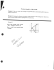### Summer Assignment 2016-17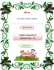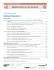### CHILDREN’S HEALTH FUND Manager of Media Relations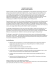### Utilizing the Hermite-Ostrogradski Formula for the Integration of Rational Functions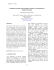### Rational decisions in non-probabilistic setting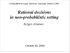### CS 188: Artificial Intelligence Course Staff Course Information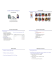### Curs 3 – 18 Octombrie 2010 Adrian Iftene [email protected]